# Today in school

There are 9 girls and 11 boys in the class today. What is the probability that Suzan will go to the board today?

p =  0.05

### Step-by-step explanation:

$p=\frac{1}{9+11}=\frac{1}{20}=0.05$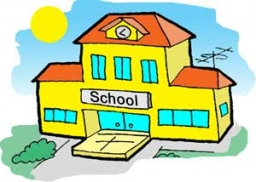Did you find an error or inaccuracy? Feel free to write us. Thank you!Tips to related online calculators
Would you like to compute count of combinations?

## Related math problems and questions:

• Boys and girlsThere are eight boys and nine girls in the class. There were six children on the trip from this class. What is the probability that left a) only boys b) just two boys
• Boys and girlsThere are 11 boys and 18 girls in the classroom. Three pupils will answer. What is the probability that two boys will be among them?
• School parliament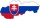There are 18 boys and 14 girls in the class. In how many ways can 3 representatives be elected to the school parliament, if these are to be: a) the boys themselves b) one boy and two girls
• Four-member teamThere are 14 girls and 11 boys in the class. How many ways can a four-member team be chosen so that there are exactly two boys in it?
• Prospering studentsThere are girls and 30 boys in the class. Boys prospering 28, girls all. How many girls are in the class and how many boys are there, if all the prospering students are 95%?
• Class - boys and girls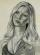In the class are 60% boys and 40% girls. Long hair has 10% boys and 80% girls. a) What is the probability that a randomly chosen person has long hair? b) The selected person has long hair. What is the probability that it is a girl?
• Two prizes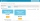In a class of 15 pupils (7 boys and 8 girls), Teacher Mae is giving away a random prize. What is probability that both prizes are won by girls?
• PupilsThere are 32 pupils in the classroom, and girls are two-thirds more than boys. a) How many percents are more girls than boys? Round the result to a whole percentage. b) How many boys are in the class? c) Find the ratio of boys and girls in the class. Writ
• Boys and girlsThere are 20 boys and 10 girls in the class. How many different dance pairs can we make of them?
• ClassroomOf the 26 pupils in the classroom, 12 boys and 14 girls, four representatives are picked to the odds of being: a) all the girls b) three girls and one boy c) there will be at least two boys
• School club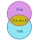Six boys and nine girls and a teacher played in the school club. What is the probability that they will point at the boy when they reproach?
• A class IV.AIn a class there are 15 girls and 30 boys.  What fraction of the class represents the boys?
• Created trioWhat is the probability that in the created trio, which consists of 19 boys and 12 girls, they will be: a) the boys themselves b) the girls themselves c) 2 boys and 1 girl?
• ClassroomThere are eighty more girls in the class than boys. Boys are 40 percent and girls are 60 percent. How many are boys and how many girls?
• Average heightThere are twice as many girls in the class as there are boys. The average height of girls is 177 cm, boys 186 cm. What is the average height of students in this class?
• Family94 boys are born per 100 girls. Determine the probability that there are two boys in a randomly selected family with three children.
• The ratioThe ratio of girls to boys in Mrs. White's class is 3:2.  If there are 12 boys, how many girls are in the class room?